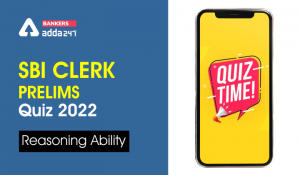Latest Banking jobs   »   10 January 2020 Sbi clerk prelims...

# 10 January 2020 Sbi clerk prelims Reasoning Daily Mock: Alphanumeric-series

SBI Clerk Exam is one of the most popular exams in the banking sector. SBI Clerk Prelims exam schedule on tentatively February/March 2020. Today we will be providing you Reasoning Quiz on- Alphanumeric Series and Order Ranking. For more preparation, you can also follow the SBI Clerk Study Plan for prelims which is provided on bankersadda.

Directions (1-5): These questions are based on the following arrangement. Study the arrangement carefully to answer these questions.
A % T 3 1 H @ 7 U O E L 8 G P # U J \$ R 2 K * C M V 9 A 6 & S Z

Q1. How many such symbols are there in the above arrangement, each of which is immediately followed by a vowel?
(a) One
(b) None
(c) Two
(d) Three
(e) More than three

Q2. Which of the following element is exactly in the middle of 3rd element from the right end and the 10th element from the left end of the arrangement?
(a) 2
(b) R
(c) \$
(d) K
(e) None of these

Q3. How many such symbols are there in the above arrangement, each of which is immediately preceded by a number and also immediately followed by a consonant?
(a) None
(b) One
(c) Two
(d) Three
(e) More than three

Q4. How many such vowels are there in the above arrangement each of which is immediately followed by a number and also immediately preceded by a consonant?
(a) None
(b) One
(c) Two
(d) Three
(e) None of these

Q5. What should come in the place of question mark (?) in the following series based on above arrangement?
OEU G8P J\$U K*?
(a) C
(b) M
(c) V
(d) 2
(e) None of these

Directions (6-8): Study the following information carefully and answer the given questions.
Among Seven friends, U, V, W, X, Y, Z and A, each got different marks in the examination. W score more marks than Y and A. V scores less marks than X. Neither X nor U score highest marks. U score more marks than Y. Neither A nor V score least. X scores more than A. The one who score second lowest marks is 70 marks. A score less marks than Y, who scores 4th highest marks. X does not score more marks than U. A does not score second lowest marks.

Q6. Who among the following score highest marks?
(a) U
(b) X
(c) W
(d) Y
(e) None of these

Q7. Who among the following person score lowest marks?
(a) A
(b) V
(c) Y
(d) W
(e) None of these

Q8. If Y scores 80 marks than what may be the score of A?
(a) 87
(b) 65
(c) 68
(d) 77
(e) 89

Q9. Inder ranked 34th from the top and 23th from the bottom among those who passed an exam. 15 boys did not participate in the exam and 9 failed in it. How many boys were there in the class?
(a) 80
(b) 75
(c) 77
(d) 81
(e) 79

Q10. Rohan is 18th from the left end of a row and Aman is 12th from the right end of row. If they interchanged their positions then Aman ranks become 20 from right end. Find total number of persons in the row?
(a) 38
(b) 37
(c) 39
(d) 36
(e) None of these

Directions (11-15): These questions are based on the following set of numbers.
978 721 327 198 654

Q11. If all the digits in each number are arranged in ascending order within the number, then which of the following will form the third highest in the new arrangement?
(a) 327
(b) 654
(c) 978
(d) 721
(e) 198

Q12. If first digit in each number is interchanged with the second digit then second digit is interchanged with third digit of number, then which of the following will form the highest number in the new arrangement?
(a) 327
(b) 654
(c) 978
(d) 721
(e) 198

Q13. What will be the difference between the second digit of the highest number and third digit of the second lowest number?
(a) 4
(b) 5
(c) 6
(d) 3
(e) None of these

Q14. If ‘2’ is added to all the given numbers, then the resultant of how many numbers will be completely divisible by 7?
(a) One
(b) Two
(c) Three
(d) Four
(e) More than four

Q15. What will be the difference of the twice of lowest number and half of the highest number?
(a) 91
(b) 87
(c) 93
(d) 95
(e) None of these

Solutions

S1.Ans.(a)
Sol. # U
S2.Ans.(b)
S3.Ans.(b)
S4.Ans.(a)
S5.Ans.(d)

Solution(6-8):
W>U>X>Y>A>V>Z

S6. Ans(c)
S7. Ans(e)
S8. Ans(d)

S9. Ans(a)
Number of boys who passed = (34 + 23 – 1) = 56
∴ Total number of boys in the class = (56 + 15 + 9) = 80

S10. Ans(b)
Total number of persons in the row=(18 + 12 – 1)=37

S11. Ans.(a)
S12. Ans.(e)
S13. Ans.(e)
S14. Ans.(b)
S15. Ans.(c)

#### If you are preparing for SBI Clerk Prelims Exam, then you can also check out a video for Reasoning below:

You may also like to read:

Practice with Crash Course and Online Test Series for SBI Clerk prelims

SBI Clerk Mock Test Online Test Series

SBI Clerk Prelims 2020 Video Course

#### Congratulations!Incorrect details? Fill the form again here

•Reasoning Ability Quiz For IBPS RRB PO P...
•Reasoning Ability Quiz For IDBI AM/Execu...
•Reasoning Ability Quiz For IBPS RRB PO P...
•Reasoning Ability Quiz For IDBI AM/Execu...
•Reasoning Ability Quiz For IBPS RRB PO P...
•Reasoning Ability Quiz For SBI Clerk Pre...